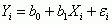# Zach’s facts

Zach’s facts have been extracted from the book to remind you of the key concepts you and Zach have learned in each chapter.

Zach's Facts 14.1 The linear model

• The linear model is a way of predicting values of one variable from another.

• We do this by fitting a statistical model to the data that summarizes the relationship between the predictor and outcome. It takes the form:• The model is defined by two types of parameters:
• b0 is called the constant or intercept. It tells us the value of the outcome when all predictor variables are zero.

• bn is called the regression coefficient for variable n. This quantifies the direction and size of the relationship between predictor n and the outcome variable.

• If bn is significantly different from 0 (i.e., where 0 means there is no relationship) then the predictor variable n significantly predicts the outcome variable. This can be established using a t-statistic and its associated p-value.
• We can assess how well the model fits the data overall using:
• R2, which tells us how much variance is explained by the model compared to how much variance there is to explain in the first place. It is the proportion of variance in the outcome variable that is shared by the predictor variable or variables.

• F, which tells us how much variability the model can explain relative to how much it can’t explain (i.e., it’s the ratio of how good the model is compared to how bad it is).

Zach's Facts 14.2 Bias in linear models

• For confidence intervals and significance tests of the linear model parameters, b, to be accurate, and for the model to generalize beyond the sample, we assume:
• Linearity: the outcome variable is, in reality, linearly related to any predictors.

• Additivity: if you have several predictors then their combined effect is best described by adding their effects together.

• Independent errors: a given error in prediction from the model should not be related to and, therefore, affected by a different error in prediction.

• Homoscedasticity: the spread of residuals is roughly equal at different points on the predictor variable.

• Normality: the sampling distribution of b and the distribution of residuals should be normal. Look at the histogram of residuals.

• There are no variables that influence the outcome that we should have included when we fit a linear model.

• All predictor variables are quantitative or dichotomous, and the outcome variable is quantitative, continuous and unbounded. All variables must have some variance.

• Multicollinearity: the relationship between two or more predictors should not be too strong. The variance inflation factors (VIFs) should be less than 10.

• The model is not influenced by specific cases. Check for residuals greater than about 3 and Cook’s distances above 1 as a sign of trouble.
• Homoscedasticity, independence of errors, and linearity are shown by a random pattern in the plot of standardized predicted values against the standardized residuals (zpred vs. zresid).

Zach's Facts 14.3 Linear models with several predictors

• Linear models can also be used to predict values of one variable from several others.

• With many predictors, the model expands to this general form:• Similar to models with one predictor:
• b0 tells us the value of the outcome when all predictor variables are zero.

• Each predictor has a parameter, b, which quantifies the direction and size of the relationship between that predictor and the outcome variable.

• If a b is significantly different from 0 (i.e., from no relationship) then the predictor variable significantly predicts the outcome variable. This can be established using a t-statistic.
• As with models with one predictor, we assess how well the model fits the data overall using R2 and F.

• The usual assumptions should be checked (Zach’s Facts 14.2).

• If assumptions are violated, bootstrap the parameters and their confidence intervals.

• For a different approach try using Bayes factors to ascertain which combination of predictors is best at predicting the outcome. The model with the largest Bayes factor is the best.